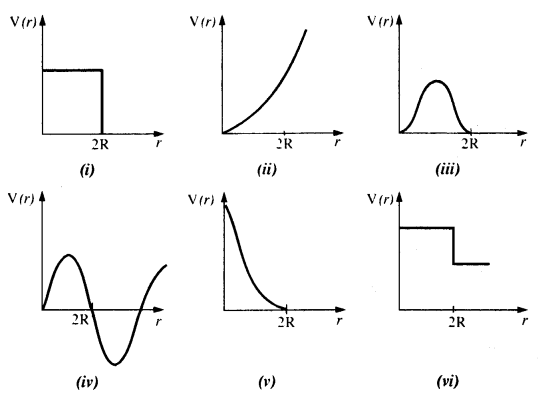## NCERT Solutions for Class 11 Physics Chapter 6 Work, Energy and Power

These Solutions are part of NCERT Solutions for Class 11 Physics. Here we have given NCERT Solutions for Class 11 Physics Chapter 6 Work, Energy and Power

Question 1.
The sign of work done by a force on a body is important to understand. State carefully if the following quantities are positive or negative:

1. work done by a man in lifting a bucket out of a well by means of a rope tied to the bucket,
2. work done by the gravitational force in the above case,
3. work done by friction on a body sliding down an inclined plane,
4. work done by an applied force on a body moving on a rough horizontal plane with uniform velocity,
5. work done by the resistive force of air on a vibrating pendulum in bringing it to rest.

1. We know that: W = $$\overrightarrow{\mathrm{F}}, \overrightarrow{\mathrm{S}}$$ = FS cos θ
‘Positive’
Reason: Force is acting in the direction of displacement (θ = 0°)
2. ’Negative’
Reason: Force is acting in the opposite direction to displacement (θ =180°)
3. ’Negative’
Reason: Force of friction is opposite to the displacement (θ = 180°)
4. ‘Positive’
Reason: The body mover in the direction of force applied (θ = 0°)
5. ‘Negative’
Reason: The resistive force opposes the motion (θ = 0°)

Question 2.
A body of mass 2 kg initially at rest moves under the action of an applied horizontal force of 7 N on a table with coefficient of kinetic friction = 0.1. Compute the
(a) work done by the applied force in 10 s
(b) work done by friction in 10 s
(c) work done by the net force on the body in 10 s
(d) change in kinetic energy of the body in 10 s and interpret your results.
Here M = 2 kg; u = 0; μ= 0.1; applied force, F = 7N, t = 10s
Force of friction, f= μMg = 0.1 x 2 x 9.8 = 1.96 N
.’. net force under which body moves, F’ = F- f = 7 – 1.96 = 5.04 N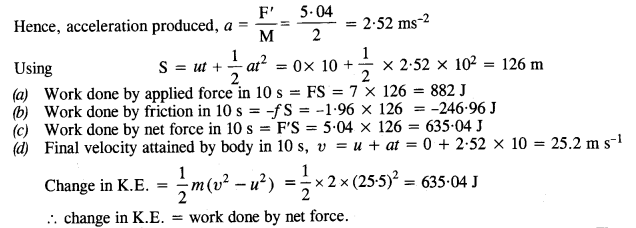Question 3.
Given in Fig. are examples of some potential energy functions in one dimension. The total energy of the particle is indicated by a cross on the ordinate axis. In each case, specify the regions, if any, in which the particle cannot be found for the given energy. Also, indicate the minimum total energy the particle must have in each case. Think of simple physical contexts for which these potential energy shapes are relevant.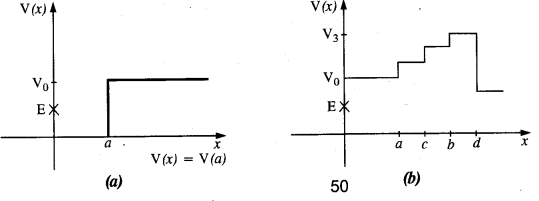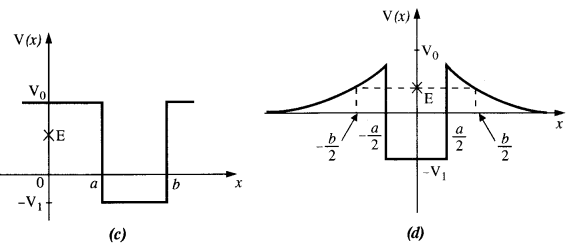(a) We know that Total energy E = KE + PE, kinetic energy can never be negative. In the region between x = 0 & x = a.
Potential energy is ‘0’. So, kinetic energy y is positive. In region x > a the potential energy has a value greater than ‘E’. So kinetic energy will be negative in this region. Hence the particle cannot be present in the region x > a.

(b) Here PE > E, the total energy of the object and as such the kinetic energy of the object would be negative. Thus object cannot be present in any region on the graph.

(c) Here x = 0 to x = a & x > b, the P E is more then E so, K E is negative. The particle cannot be present in these portions.

(d) The object cannot exist in the region between
x = $$\frac{-b}{2}$$ to x = $$\frac{-a}{2}$$ & x = $$\frac{-a}{2}$$ to x = $$\frac{-b}{2}$$
Because in this region P E > E.

Question 4.
The potential energy function for a particle executing linear simple harmonic motion is given by V (x) = Kx/2, where A is the force constant of the oscillator. For A = 0.5 N nr1, the graph of V (x) versus x is shown in Fig. Show that a particle of total energy 1 J moving under this potential must ‘turn back’ when it reaches x = ± 2 m.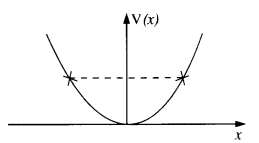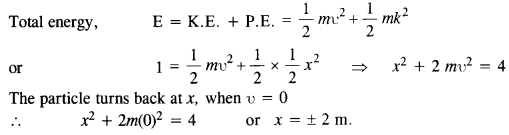Question 5.
(a) The casing of a rocket in flight burns up due to friction. At whose expense is the heat energy required for burning obtained? The rocket or the atmosphere ?
(b) Comets move around the sun in highly elliptical orbits. The gravitational force on the comet due to the sun is not normal to the comet’s velocity in general. Yet the work done by the gravitational force over every complete orbit of the comet is zero. Why?
(c) An artificial satellite orbiting the earth in very thin atmosphere loses its energy gradually due to dissipation against atmospheric resistance, however small. Why then does its speed increase progressively as it comes closer and closer to the earth ?
(d) In Fig.

1. The man walks 2 m carrying a mass of 15 kg on his hands. In Fig.
2. He walks the same distance pulling the rope behind him. The rope goes over a, pulley, and a mass of 15 kg hangs at its other end. In which case is the work done greater ?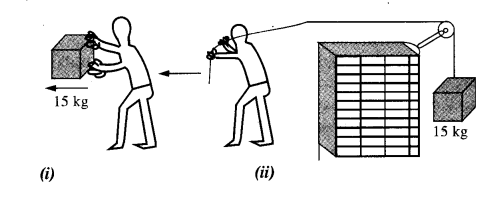(a) Heat energy required for the burning of the casing of a rocket is obtained by a rocket. Since the work is done against the friction, the kinetic energy of the rocket decreases continuously and this work against friction reappears as heat energy.

(b) This is because of the conservative nature of the gravitational force. Work done by the gravitational force in a closed path is zero.

(c) As an artificial satellite gradually loses its energy due to dissipation against atmospheric resistance, its potential decreases rapidly. As a result, the kinetic energy of the satellite slightly increases i.e. its speed increases progressively.

(d) In fig (i) the force applied by the man is perpendicular to the direction of movement of mass, i.e. θ = 90°
W = Fs cos θ = Fs cos 90° = 0
In figure (ii) the force applied is in direction of the movement of mass i.e. θ = o°
∴ W = Fs cos θ
= Mgs cos θ
= 15 × 9.8 × 2 × 1
= 294 J.

Question 6.
Underline the correct alternative:
(a)
When a conservative force does positive work on a body, the potential energy of the body increases/decreases/ remains unaltered.
(b) Work done by a body against friction always results in a loss of its kinetic/potential energy.
The rate of change of total momentum of many-particle system is proportional to the external force/sum of the internal forces on the system.
(c) In an inelastic collision of two bodies, the quantities which do not change after the collision are the total kinetic energy total linear momentum!total energy of the system of two bodies.
(a) Work done by conservative force is equal to the negative of potential energy. When work done is positive, potential energy decreases.
(b)  Kinetic energy, because friction does work against motion of the body.
(c) External force, because in many-particle systems, the internal forces in the system cancel each other and hence cannot change the net momentum of the system.
(d) In inelastic collision, total energy and linear momentum are conserved. However, kinetic energy decreases.

Question 7.
State if each of the following statements is true or false. Give reasons for your answer.

1. In an elastic collision of two bodies, the momentum and energy of each body are conserved.
2. The total energy of a system is always conserved, no matter what internal and external forces on the body are present.
3. Work done in the motion of a body over a closed loop is zero for every force in nature.
4. In an inelastic collision, the final kinetic energy is always less than the initial kinetic energy of the system.

1. False, the momentum and energy of each body are conserved.
2. False, the external force on the system may increase or decrease the total energy of the system.
3. False, for the nonconservative forces (friction) the work done in closed-loop is not zero.
4. True, usually in an inelastic collision the final kinetic energy is always less than the initial kinetic energy of the system.

Question 8.
(a) In an elastic collision of two billiard balls, is the total kinetic energy conserved during the short time of collision of the balls (i.e., when they are in contact) ?
(b) Is the total linear momentum conserved during the short time of an elastic collision of two balls ?
(c) What are the answers to (a) and (b) for an inelastic collision ?
(d) If the potential energy of two billiard balls depends only on the separation distance between their centers, is the collision elastic or inelastic ? (Note, we are talking here of potential energy corresponding to the force during collision, not gravitational potential energy).
(a) In this case total kinetic energy is not conserved because when the bodies are in contact during elastic collision even, the kinetic energy is converted into internal energy.
(b) Yes, because total momentum conserves as per law of conservation of momentum.
(c) In inelastic collision, K.E. is not conserved but linear momentum is conserved.
(d) It is a case of elastic collision because in this case the forces are of conservative nature

Question 9.
A body is initially at rest. It undergoes one-dimensional motion with constant acceleration. The power delivered to it at time t is proportional to
(1) tm
(2) t
(3) t3/2
(4) t2Question 10.
A body is moving uni-directionally under the influence of a source of constant power. Its displacement in time t is proportional to
(1) tm
(2) t
(3) t3/2
(4) f2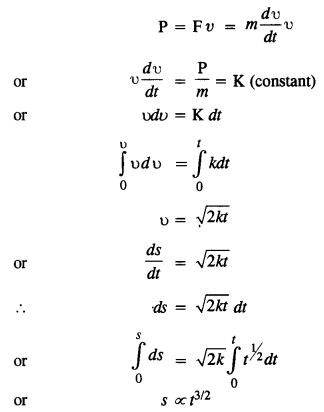Question 11.
A body constrained to move along the z-axis of a coordinate system is subject to a constant force F given by $$\vec { F }=$$ $$\hat {i}+{ 2j }+{ 3K }$$
where $$\hat {i}+{ j }+{ K }$$are unit vectors along the x-, y- and z-axis of the system respectively. What is the work done by this force in moving the body a distance of 4 m along the z-axis ?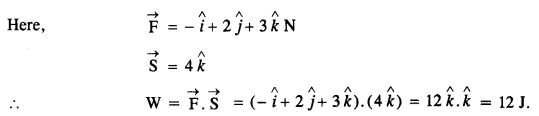Question 12.
An electron and a proton are detected in a cosmic ray experiment, the first with kinetic energy 10 keV, and the second with 100 keV. Which is faster, the electron or the proton? Obtain the ratio of their speeds, (electron mass = 9.11 x 10-31 kg, proton mass = l.67 x 10-27 kg, 1 eV = 1.60 x 10-19 J).
Let υe = Speed of electron
υp = speed of the proton
mg = mass of electron
and mp = mass of proton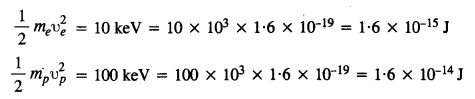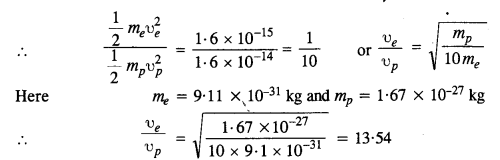Question 13.
A rain drop of radius 2 mm falls from a height of 500 m above the ground. It falls with decreasing acceleration (due to viscous resistance of the air) until at half its original height, it attains its maximum (terminal) speed, and moves with uniform speed thereafter. What is the work done by the gravitational force on the drop in the first and second half of its journey ? What is the work done by the resistive force in the entire journey if its speed on reaching the ground is 10 ms-1?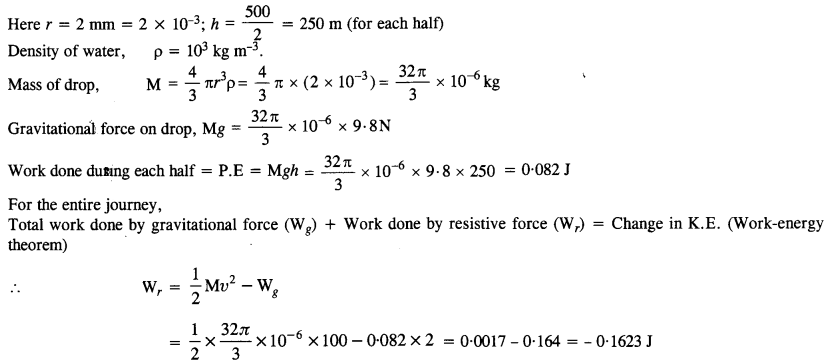Question 14.
A molecule in a gas container hits a horizontal wall with speed 200 ms-1 and angle 30° with the normal, and rebounds with the same speed. Is momentum conserved in the collision ? Is the collision elastic or inelastic ?
In all types of collisions, momentum is conserved. Let us check the conservation of kinetic energy.As the wall is too heavy, the recoiling molecule produces no velocity in the wall. l%m is mass of the gas molecule and M is mass of wall, then total K.E. after collision,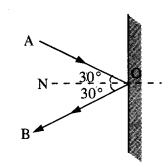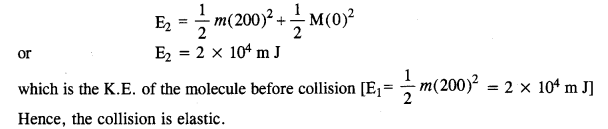Question 15.
A pump on the ground floor of a building can pump up water to fill a tank of volume 30 m3 in 15 min. If the tank is 40 m above the ground, and the efficiency of the pump is 30%, how much electric power is consumed by the pump ?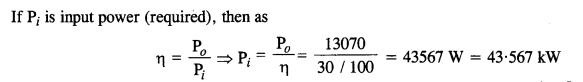Question 16.
Two identical ball bearings in contact with each other and resting on a frictionless. table are hit head-on by another ball bearing of the same mass moving initially with a speed V. If the collision is elastic, which of the following (Fig.) is a possible result after collision ?Collision is elastic, so K.E. of the system is conserved.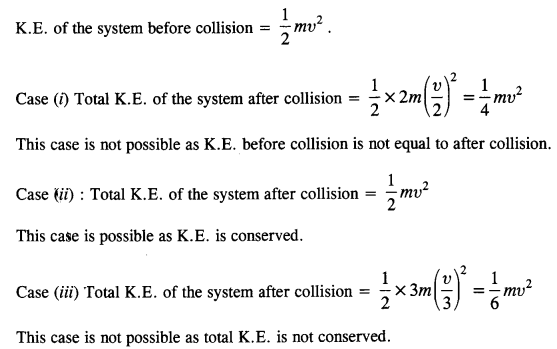Question 17.
The bob A of a pendulum released from 30° to the vertical hits another bob B of the same mass at rest on a table as shown in Fig. How high does the bob A rise after the collision? Neglect the size of the bobs and assume the collision to be elastic.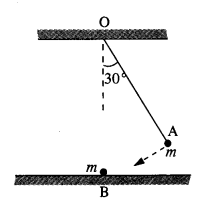Since the collision is elastic, therefore A would come to rest and B would begin to move with the velocity of A to conserve the linear momentum.

Question 18.
The bob of a pendulum is released from a horizontal position A as shown in Fig. If the length of the pendulum is 1.5 m, what is the speed with which the bob arrives at the lowermost point B, given that it dissipated 5% of its initial energy against air resistance ?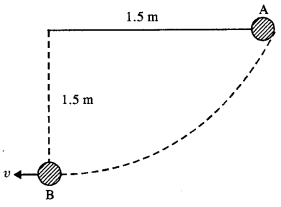P.E. of the bob at position A = mgh = m x 9.8 x 1.5
Since 5% of energy is lost when reach at B, so K.E. at the lowermost point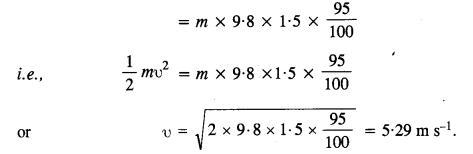Question 19.
A trolley of mass 300 kg carrying a sandbag of 25 kg is moving uniformly with a speed of 27 km/h on a frictionless track. After a while, sand starts leaking out of a hole on the trolley’s floor at the rate of 0.05 kgs_1. What is the speed of the trolley after the entire sandbag is empty ?
The system of trolley and sandbag is moving at a uniform speed. Clearly, the system is not being acted upon by external force. If the sand leaks out, even when no external force acts. So there shall no change in the speed of the trolley.

Question 20.
A particle of mass 0.5 kg travels in a straight line with velocity υ = ax3/2, where a = 5 m-1/2 s-1. What is the work done by the net force during its displacement from x = 0 to x = 2m?
Here m = 0.5 kgQuestion 21.
The blades of a windmill sweep out a circle of area A.
(a) If the wind flows at a velocity v perpendicular to the circle, what is the mass of the air passing through it in time t ?
(b) What is the kinetic energy of the air ?
(c) Assume that the windmill converts 25% of the wind’s energy into electrical energy, and that A = 30 m2, υ= 36 km/h and the density of air is 1.2 kg m-3. What is the electrical power produced ?
(a) Volume of wind flowing per sec = Aυ
Mass of wind flowing per sec = Aυp
Mass of air passing in time t = AυptQuestion 22.
A person trying to lose weight (dieter) lifts a 10 kg mass 0.5 m, 1000 times. Assume that the potential energy lost each time she lowers the mass is dissipated,
(a) How much work does she do against the gravitational force ?
(b) Fat supplies 3.8 x 107 J of energy per kilogram which is converted to mechanical energy with a 20% efficiency rate. How much fat will the dieter use up ?
Here m = 10 kg, h = 0.5 m, n = 1000
(a) Work done against gravitational force = W = n mgh
= 1000 x (10 x 9.8 x 0.5)
= 49000 J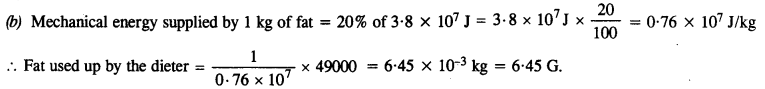Question 23.
A large family uses 8 kW of power,
(a) Direct solar energy is incident on the horizontal surface at an average rate of 200 W per square meter. If 20% of this energy can be converted to useful electrical energy, how large an area is needed to supply 8 kW?
(b) Compare this area to that of the roof of a house constructed on a plot of size 20 m x 15 m with a permission to cover upto 70%.
(a) Energy incident per square meter = 200 W
Let A be the area needed to supply 8 kW
.’. Energy incident on area A = (200 A) W
Energy converted into useful electrical energy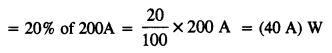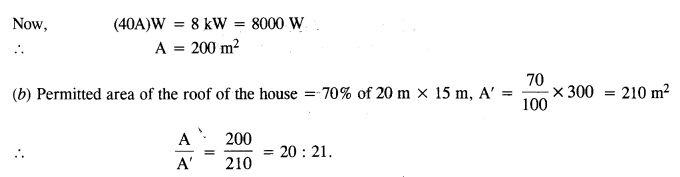Question 24.
A bullet of mass 0.012 kg and horizontal speed 70 ms-1 strikes a block of wood of mass 0.4 kg and instantly comes to rest with respect to the block. The block is suspended from the ceding by means of thin wires. Calculate the height to which the block rises. Also, estimate the amount of heat produced in the block.
By using law of conservation of momentum,
m1u1+ m2u2 = (m1 +m2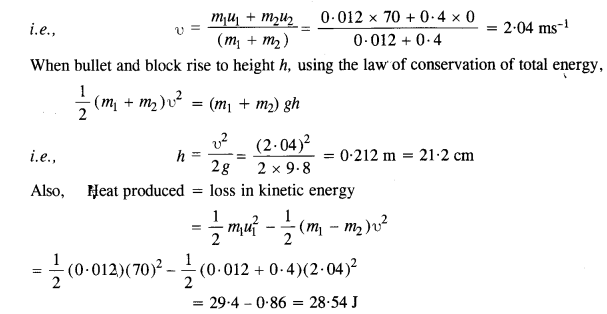Question 25.
Two inclined frictionless tracks, one gradual and the other steep meet at A from where two stones are allowed to slide down from rest, one on each track (Fig.). Will the stones reach the bottom at the same time ? Will they reach there with the same speed ? Explain. Given θ1 = 30°,θ2 = 60°, and h = 10 m, what are the speeds and times taken by the two stones?Here, K.E. at bottom = P.E. at topSince vertical height of both planes is same, so they will reach the bottom with same speed. Acceleration of a body sliding down an inclined plane, a = g sin θ
Let t be the time taken by stone 1 to travel AB distance.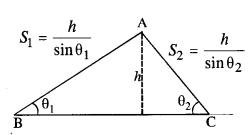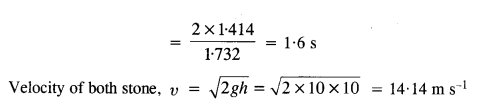Question 26.
A 1 kg block situated on a rough incline is connected to a spring of spring constant 100 N m-1 as shown in Fig. The block is released from rest with the spring in the unstretched position. The block moves 10 cm down the incline before coming to rest. Find the coefficient of friction between the block and the incline. Assume that the spring has negligible mass and the pulley is frictionless.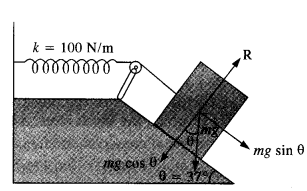From fig, R = mg cos θ
Force of friction, F = μR = μ mg cosθ
Net force on the block down the incline
= mg sinθ – F = mg sinθ – (a mg cosθ = mg (sinθ – μcos θ)
Distance moved, x = 10 cm = 0.1 m
In equilibrium, Work done = P.E. of stretched spring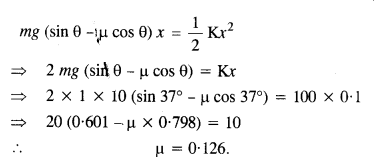Question 27.
A bolt of mass 0.3 kg falls from the ceiling of an elevator moving down with a uniform speed of 7 ms-1. It hits the floor of the elevator (length of the elevator = 3 m) and does not rebound. What is the heat produced by the impact ? Would your answer be different if the elevator were stationary ?
Potential energy of bolt = mgh = 0.3 × 9.8 × 3 = 8.82 J.
Since the bolt does not rebound, the while energy is converted into heat. Since the value of acceleration due to gravity is the same in all inertial systems, the answer will not change even if the elevator is stationary.

Question 28.
A trolley of mass 200 kg moves with a uniform speed of 36 km/h on a frictionless track. A child of mass 20 kg bins on the trolley from one end to the other (10 m away) with a speed of 4 ms-1 relative to the trolley in a direction opposite to the trolley’s motion and jumps out of the trolley. What is the final speed of the trolley ? How much has the trolley moved from the time the child begins to run ?
Initial total momentum = pi = (m1 + m2) u1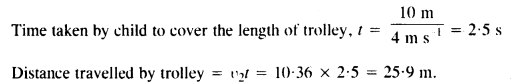Question 29.
Which of the following potential energy curves in Fig. cannot possibly describe the elastic collision of two billiard balls ? Here r is the distance between centers of the balls.